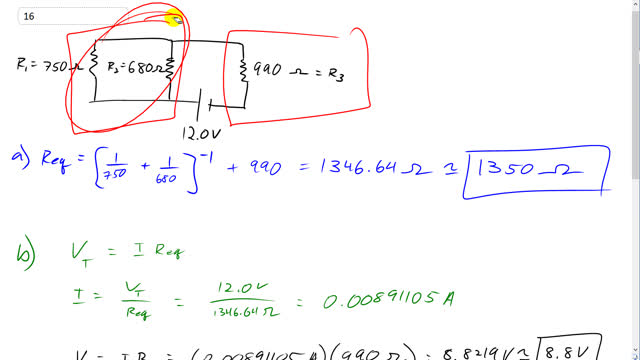## You are here

Question:

Determine

1. the equivalent resistance of the circuit shown in Fig. 19–48,
2. the voltage across each resistor, and
3. the current through each resistor.Figure 19-48.

Giancoli, Douglas C., Physics: Principles with Applications, 7th Ed., ©2014. Reprinted by permission of Pearson Education Inc., New York.
The question will be visible after logging in, as required by Pearson Education Inc.

1. $1350 \; \Omega$
2. $V_3 = 8.8 \textrm{ V, } V_1 = V_2 = 3.2 \textrm{ V}$
3. $I_3 = 0.0089 \textrm{ A, } I_2 = 0.0047 \textrm{ A, } I_1 = 0.0042 \textrm{ A}$

# Giancoli 7th Edition, Chapter 19, Problem 16

(2:16)Hi Agp2196, $R_1$ and $R_2$ can be recognized as in parallel since they have both ends connected by wires. The bottom ends are connected by a wire, and the top ends are also connected by a wire. This means current at the bottom, coming from the positive end of the battery, has a choice of $R_1$ or $R_2$, and when you see current being given this choice, you know the resistors are in parallel. Resistor labelled $R_3$ is in series since there are no other options but to go through $R_3$ in order to continue through the circuit.